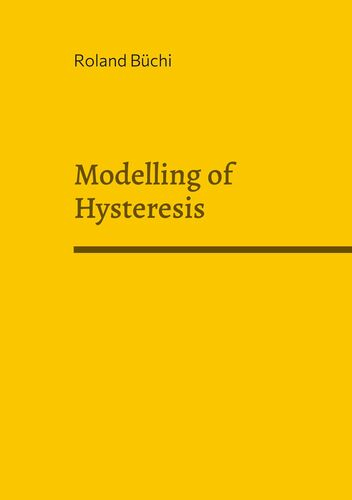# Modelling of Hysteresis

Industrie & Technik

Paperback

28 Seiten

ISBN-13: 9783756293698

Verlag: Books on Demand

Erscheinungsdatum: 11.08.2022

Sprache: Englisch

Farbe: Nein

Bewertung::
0%
CHF 13.50

inkl. MwSt. / portofrei

sofort verfügbar

Ihr eigenes Buch!

Werden Sie Autor*in mit BoD und erfüllen Sie sich den Traum vom eigenen Buch und E-Book.

Mehr erfahren
In order to model a real system, differential equations are usually derived, which are based on physical principles. When modelling a characteristic hysteresis, the mathematical equations are also based on the physics underlying hysteresis, but they are nonlinear effects. Therefore, a different approach is taken in the modelling. A measurement is used as the basis for modelling. This means that a specific measurement curve that determines the parameters must first be available. The advantage of this method is that it can be applied to many technical fields. The method presented here can be verified using the example of piezo elements, but it can also be applied to the magnetization characteristic of ferromagnetic materials. The methods of Preisach and Mayergoyz are presented and explained in this booklet. Examples are calculated with Matlab. The Matlab source code is printed at the end.Roland Büchi

Es sind momentan noch keine Pressestimmen vorhanden.

Eigene Bewertung schreiben
Bitte melden Sie sich hier an, um eine Rezension abzugeben.